2022 NL Cy Young Odds
+350
3.5 to 1Corbin Burnes
15.0% implied probability

+700
7 to 1Carlos Rodon
8.5% implied probability

+900
9 to 1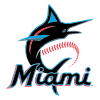Pablo Lopez
6.8% implied probability

+1000
10 to 1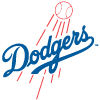Walker Buehler
6.1% implied probability

+1200
12 to 1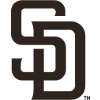Joe Musgrove
5.2% implied probability

+1700
17 to 1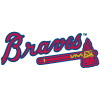Max Fried
3.8% implied probability

+2000
20 to 1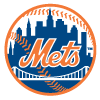Max Scherzer
3.2% implied probability

+2000
20 to 1Zac Gallen
3.2% implied probability

+2000
20 to 1Zack Wheeler
3.2% implied probability

+2000
20 to 1Sandy Alcantara
3.2% implied probability

+2500
25 to 1Brandon Woodruff
2.6% implied probability

+3000
30 to 1Chris Bassitt
2.2% implied probability

+3000
30 to 1Aaron Nola
2.2% implied probability

+3000
30 to 1Clayton Kershaw
2.2% implied probability

+3500
35 to 1Logan Webb
1.9% implied probability

+3500
35 to 1Kyle Wright
1.9% implied probability

+4000
40 to 1Julio Urias
1.6% implied probability

+4000
40 to 1Eric Lauer
1.6% implied probability

+5000
50 to 1Sean Manaea
1.3% implied probability

+5000
50 to 1Freddy Peralta
1.3% implied probability

+5000
50 to 1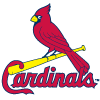Miles Mikolas
1.3% implied probability

+6600
66 to 1Josh Hader
1.0% implied probability

+8000
80 to 1Alex Cobb
0.8% implied probability

+8000
80 to 1Yu Darvish
0.8% implied probability

+8000
80 to 1Tylor Megill
0.8% implied probability

+8000
80 to 1Jacob deGrom
0.8% implied probability

+8000
80 to 1Trevor Rogers
0.8% implied probability

+8000
80 to 1Kyle Gibson
0.8% implied probability

+10000
100 to 1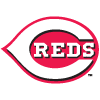Luis Castillo
0.7% implied probability

+10000
100 to 1Ranger Suarez
0.7% implied probability

+10000
100 to 1Ian Anderson
0.7% implied probability

+10000
100 to 1Edwin Diaz
0.7% implied probability

+10000
100 to 1Adam Wainwright
0.7% implied probability

+10000
100 to 1Tyler Mahle
0.7% implied probability

+10000
100 to 1Anthony DeSclafani
0.7% implied probability

+10000
100 to 1Carlos Carrasco
0.7% implied probability

+10000
100 to 1Adrian Houser
0.7% implied probability

+10000
100 to 1Andrew Heaney
0.7% implied probability

+10000
100 to 1Alex Wood
0.7% implied probability

+10000
100 to 1Merrill Kelly
0.7% implied probability

+10000
100 to 1Mike Clevinger
0.7% implied probability

+10000
100 to 1Charlie Morton
0.7% implied probability

+12500
125 to 1Jack Flaherty
0.5% implied probability

+12500
125 to 1Jesus Luzardo
0.5% implied probability

+12500
125 to 1German Marquez
0.5% implied probability

+15000
150 to 1Madison Bumgarner
0.4% implied probability

+15000
150 to 1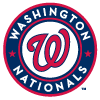Stephen Strasburg
0.4% implied probability

+15000
150 to 1Blake Snell
0.4% implied probability

+20000
200 to 1Josiah Gray
0.3% implied probability

+20000
200 to 1Dustin May
0.3% implied probability

+20000
200 to 1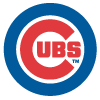Kyle Hendricks
0.3% implied probability

+20000
200 to 1Mike Soroka
0.3% implied probability

+20000
200 to 1Wade Miley
0.3% implied probability

+20000
200 to 1Dinelson Lamet
0.3% implied probability

+20000
200 to 1Adbert Alzolay
0.3% implied probability

+25000
250 to 1Marcus Stroman
0.3% implied probability

+25000
250 to 1Mitch Keller
0.3% implied probability

+30000
300 to 1Steven Matz
0.2% implied probability

+50000
500 to 1Patrick Corbin
0.1% implied probability